# QlikView App Dev

Discussion Board for collaboration related to QlikView App Development.

Announcements
Now Live: Qlik Sense SaaS Simplified Authoring – Analytics Creation for Everyone: READ DETAILS
cancel
Showing results for
Did you mean: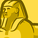Creator

## How to create buckets using percentage value

Hi,

Please help me to create below buckets using % to Goal values. And also how to calculate count of Sales Rep for each bucket.

% to Goal - Sum(Sales)/Sum(PY Sales)

Buckets:

<=95% -if  % to Goal <=0.95

95%-97% - if % to Goal >0.95 and <=0.97

98%-99% - if % to Goal >0.97 and <=0.99

>100%% to Goal >=1

Using the above bucket i want to calculate count of Sales rep for each bucket as below

Count of Sales Rep:

<=95% - 18

95%-97% - 5

98%-99% - 5

>100% - 37

Please help me to create a bar chart with above buckets and count of sales rep as below. In the below bar graph instead of 65,65,52,51 i want to get values as 18,5,5, 37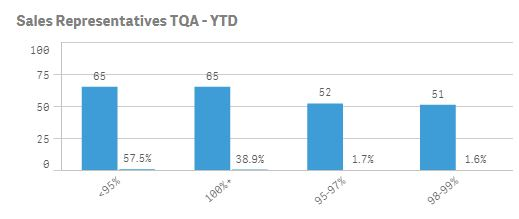1 Solution

Accepted SolutionsMVP

I got close to what you need, but not exact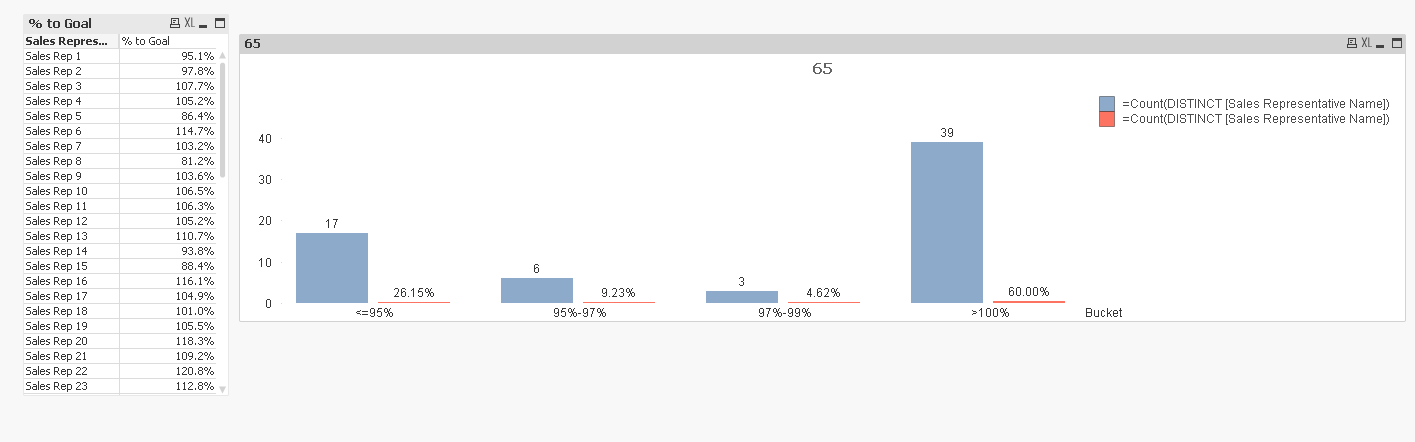9 RepliesCreator II

Where you need to creates this buckets it's in script or front end chart.

AAKMVP

I got close to what you need, but not exactMaster

Hi Ayessha,

I changed your bucket definition a little because there was no bucket for >99%<100%.

Got this chart: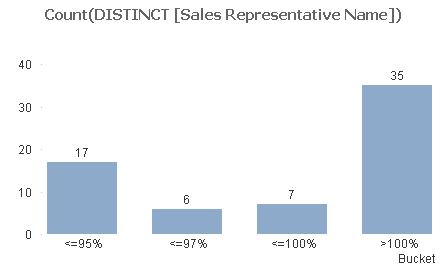The Bucket dimension is:

=Aggr(

Pick(

Match(-1,

Sum (Sales)/Sum (Goal)<=0.95,

Sum (Sales)/Sum (Goal)<=0.97,

Sum (Sales)/Sum (Goal)<=1,

-1),

'<=95%',

'<=97%',

'<=100%',

'>100%')

,[Sales Representative Name])

Regards

Andrew

PS

Sort by expression Sum (Sales)/Sum (Goal)Creator
Author

Thank you so much sunny. It is exactly what i wanted.Creator
Author

Thank you so much Andrew. Even your answer is correct. Unfortunately i cannot mark it as correct. Hence marked it as helpful.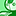Contributor

Hi Sunny,

Can you please post the Solution in text file as i am using Qlik Sense and i do not have access to Qlik View for viewing file. Any help would be appreciated. Thanks,

Regards,

ShaunakContributor

Hi Andrew,

Can you please explain this snippet, especially what does the -1 do in the above script?

Regards,

ShaunakMaster

Hi, Sorry for the late reply.  -1 means TRUE.

Suppose Sum (Sales)/Sum (Goal) = 0.96, so "Sum (Sales)/Sum (Goal)<=0.97" is the first true statement inside MATCH.

Match(-1,

Sum (Sales)/Sum (Goal)<=0.95,

Sum (Sales)/Sum (Goal)<=0.97,

Sum (Sales)/Sum (Goal)<=1,

-1)

Evaluating "Sum (Sales)/Sum (Goal)<=0.95" will return 0. Because in this case that statement is not true. 0 does not match -1 so the MATCH statement moves on to the next line. The next line is true, will return -1 and will match the first argument of the MATCH statement so the MATCH statement will return 2.

The final -1 is there to catch any examples where Sum (Sales)/Sum (Goal)>1 because if Sum (Sales)/Sum (Goal)>1 the MATCH statement will MATCH -1 to -1 and return 4.

Kind regards

AndrewContributor

Hi Andrew,

Thanks for your reply and really appreciate your efforts for explaining the snippet to me,it was helpful to me in a live scenario. Thanks a lot!!

Regards,

Shaunak